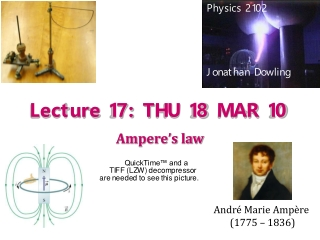DownloadDownload PresentationLecture 17: THU 18 MAR 10

# Lecture 17: THU 18 MAR 10

Télécharger la présentation## Lecture 17: THU 18 MAR 10

- - - - - - - - - - - - - - - - - - - - - - - - - - - E N D - - - - - - - - - - - - - - - - - - - - - - - - - - -
##### Presentation Transcript

1. Physics 2102 Jonathan Dowling Lecture 17: THU 18 MAR 10 Ampere’s law André Marie Ampère (1775 – 1836)

2. q q Flux = 0! Remember Gauss Law for E-Fields? Given an arbitrary closed surface, the electric flux through it is proportional to the charge enclosed by the surface.

3. Gauss’s Law for B-Fields! No isolated magnetic poles! The magnetic flux through any closed “Gaussian surface” will be ZERO. This is one of the four “Maxwell’s equations”. There are no SINKS or SOURCES of B-Fields! What Goes IN Must Come OUT!

4. i4 i3 i2 i1 ds Ampere’s law: Closed Loops The circulation of B (the integral of B scalar ds) along an imaginary closed loop is proportional to the net amount of current traversing the loop. Thumb rule for sign; ignore i4 If you have a lot of symmetry, knowing the circulation of B allows you to know B.

5. Ampere’s law: Closed Loops The circulation of B (the integral of B scalar ds) along an imaginary closed loop is proportional to the net amount of current traversing the loop. Thumb rule for sign; ignore i3 If you have a lot of symmetry, knowing the circulation of B allows you to know B.

6. C a l c u l a t i o n o f i . W e c u r l t h e f i n g e r s e n c o f t h e r i g h t h a n d i n t h e d i r e c t i o n i n w h i c h t h e A m p e r i a n l o o p w a s t r a v e r s e d . W e n o t e t h e d i r e c t i o n o f t h e t h u m b .

7. Sample Problem • Two square conducting loops carry currents of 5.0 and 3.0 A as shown in Fig. 30-60. What’s the value of ∫B∙dsthrough each of the paths shown? Path 1: ∫B∙ds = •(–5.0A+3.0A) Path 2: ∫B∙ds = •(–5.0A–5.0A–3.0A)

8. R Ampere’s Law: Example 1 • Infinitely long straight wire with current i. • Symmetry: magnetic field consists of circular loops centered around wire. • So: choose a circular loop C so B is tangential to the loop everywhere! • Angle between B and ds is 0. (Go around loop in same direction as B field lines!) Much Easier Way to Get B-Field Around A Wire: No Calculus.

9. Ampere’s Law: Example 2 i Current out of page, circular field lines • Infinitely long cylindrical wire of finite radius R carries a total current i with uniform current density • Compute the magnetic field at a distance rfrom cylinder axisfor: r < a (inside the wire) r > a (outside the wire)

10. Ampere’s Law: Example 2 (cont) Current out of page, field tangent to the closed amperian loop Need Current Density J! For r>R, ienc=i, so B=m0i/2pR = LONG WIRE! For r < R

11. B O R r Ampere’s Law: Example 2 (cont)

12. Solenoids The n = N/L is turns per unit length.

13. Magnetic Field in a Toroid “Doughnut” Solenoid Toriod Fusion Reactor: Power NYC For a Day on a Glass of H2O

14. All loops in the figure have radius r or 2r. Which of these arrangements produce the largest magnetic field at the point indicated? Magnetic Field of a Magnetic Dipole A circular loop or a coil currying electrical current is a magnetic dipole, with magnetic dipole moment of magnitude =NiA. Since the coil curries a current, it produces a magnetic field, that can be calculated using Biot-Savart’s law:

15. Magnetic Dipole in a B-Field Force is zero in homogenous field Torque is maximum when = 90° Torque is minimum when = 0° or 180° Energy is maximum when = 180° Energy is minimium when = 0°

16. Neutron Star a Large Magnetic Dipole